# Problems

Оценка 4.9
doc
08.05.2020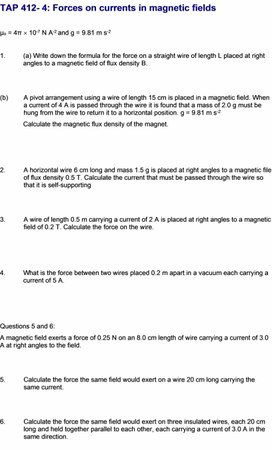problems.doc

TAP 412- 4: Forces on currents in magnetic fields

µo = 4π ´ 10-7 N A-2 and g = 9.81 m s-2

1.         (a) Write down the formula for the force on a straight wire of length L placed at right         angles to a magnetic field of flux density B.

(b)        A pivot arrangement using a wire of length 15 cm is placed in a magnetic field. When a current of 4 A is passed through the wire it is found that a mass of 2.0 g must be hung from the wire to return it to a horizontal position. g = 9.81 m s-2

Calculate the magnetic flux density of the magnet.

2.         A horizontal wire 6 cm long and mass 1.5 g is placed at right angles to a magnetic file of flux density 0.5 T. Calculate the current that must be passed through the wire so that it is self-supporting

3.         A wire of length 0.5 m carrying a current of 2 A is placed at right angles to a magnetic field of 0.2 T. Calculate the force on the wire.

4.         What is the force between two wires placed 0.2 m apart in a vacuum each carrying a        current of 5 A.

Questions 5 and 6:

A magnetic field exerts a force of 0.25 N on an 8.0 cm length of wire carrying a current of 3.0 A at right angles to the field.

5.         Calculate the force the same field would exert on a wire 20 cm long carrying the same current.

6.         Calculate the force the same field would exert on three insulated wires, each 20 cm long and held together parallel to each other, each carrying a current of 3.0 A in the same direction.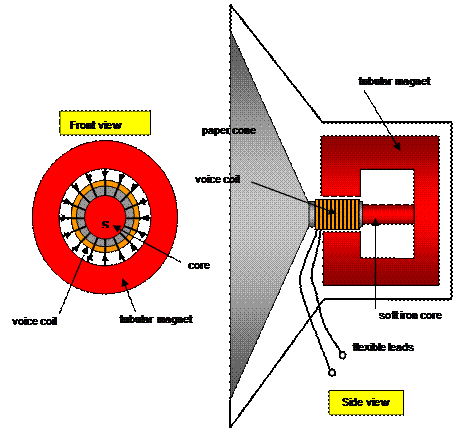7. (a) Using the ideas of forces on currents in magnetic fields and the diagram explain the operation of a moving coil loudspeaker.

(b) Calculate the force on the voice coil of a loudspeaker that has a diameter of 2 cm, a 100 turns and carries a current of 20 mA if the field strength of the tubular magnet is 0.1T.

Answers and worked solutions

1(a)      F = BIL

(b)      B=F/IL

B = [2.0 x 10-3 x 9.81]/ [4 x 0.15] = 0.0327 T (Remember to change grams to kg and centimetres to metres)

2          Weight of mass required = BIL

Therefore: 1.5 x 10-3 x 9.81 = 0.5 x I x 0.06 giving I = 0.49 A

3          F = BIL = 0.2 x 2 x 0.5 = 0.2 N

4          F = moI2L/2pr = 4p x10-7 x 25 x ½ p x 0.2 = 2.5 x10-5 N

5          For 20 cm length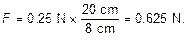6.         If each wire carries 3.0 A this is the same as effective current of 9.0 A,

so force F = 3 ´ 0.625 = 1.9 N.

7(a)      The wire of voice coil is at right angles to the field. When a varying current from an amplifier current flows in the coil it experiences a force that moves the coil backwards and forwards along the soft iron core.

(b)      F = nBIL = 100 x 0.1 x 20 x 10-3 x p x 2 x 10-2 = 0.013 N

(Note: cm to m and mA to A)

External references

Questions 1-4 and 7 are taken from Resourceful Physics Questions 5 and 6 are from Advancing Physics chapter 15, 250S

## TAP 412- 4: Forces on currents in magnetic fields µ o = 4π ´ 10 -7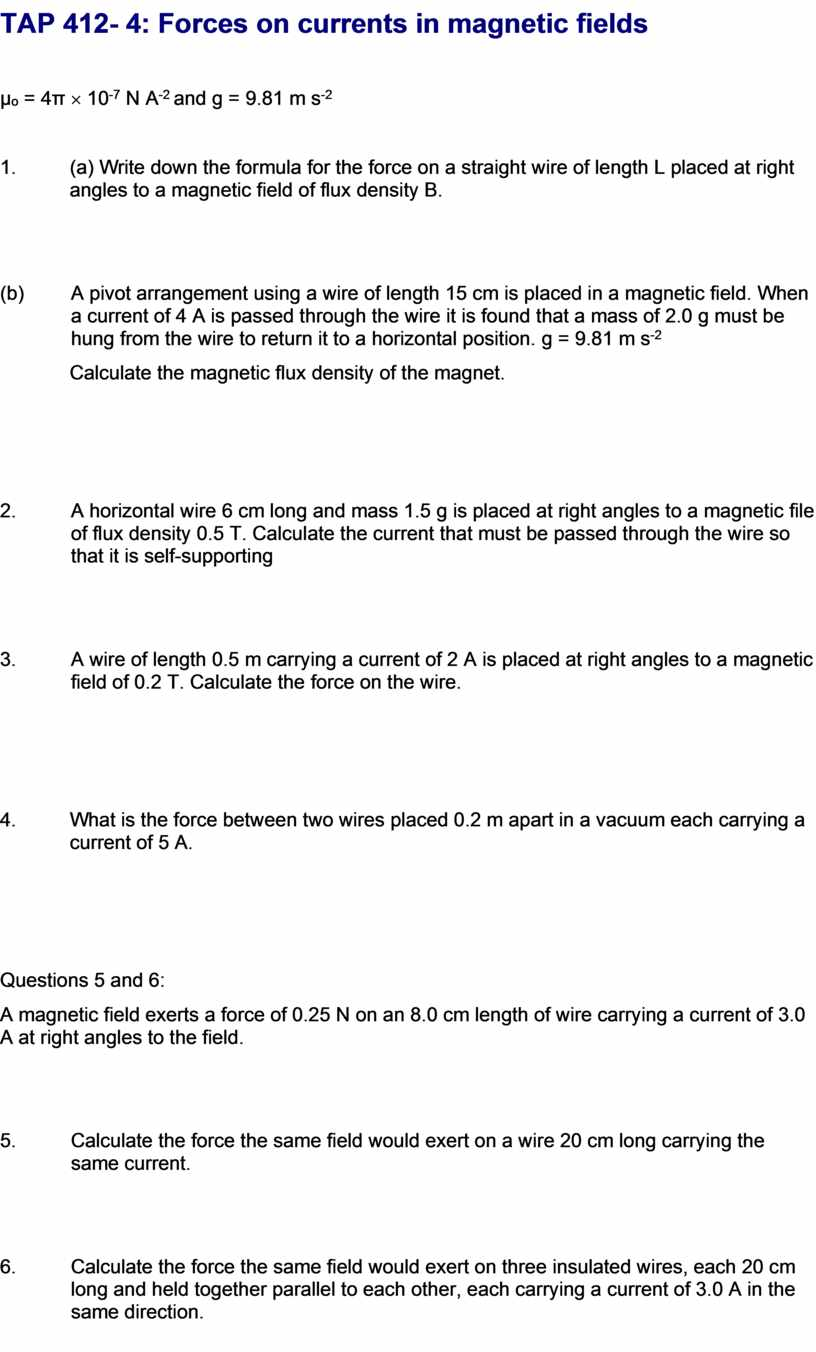## Using the ideas of forces on currents in magnetic fields and the diagram explain the operation of a moving coil loudspeaker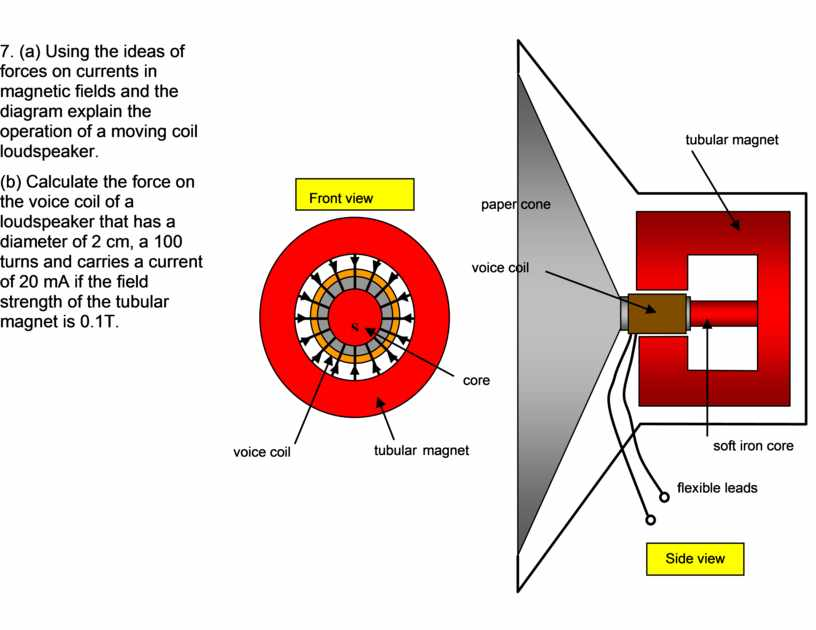Скачать файл